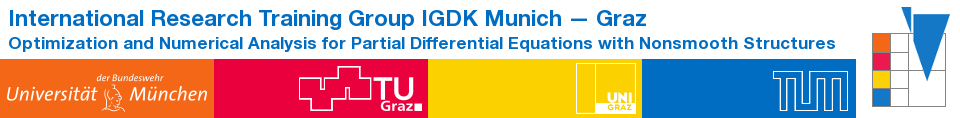IGDK1754QualificationProgramCompactCoursesCCHerzog2013

An Introduction to PDE-Constrained Optimization

Prof. Dr. Roland Herzog

Abstract

The term 'PDE-constrained optimization' refers to mathematical optimization problems in which partial differential equations (PDEs) appear as equality constraints. This problem class comprises optimal control, shape optimization, and parameter identification problems.

In this introductory course we begin by reviewing fundamental concepts, in particular optimality conditions, for finite dimensional optimization problems. We will then focus on prototypical problems in PDE-constrained optimization and develop their optimality conditions. Finally, we will provide a brief overview over algorithmic paradigms.

Date and Place